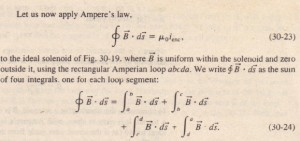# Solenoids and Toroids

Solenoids and Toroids

Magnetic Field of a Solenoid

We now turn our attention to another situation in which Ampere’s law proves useful. It concerns the magnetic field produced by the current in a long, tightly wound helical coil of wire. Such a coil is called a solenoid . We assume that the length of the solenoid is much greatt:r than the diameter.  shows a section through a portion of a “stretched-out” solenoid. The solenoid’s magnetic field is the vector sum of the fields produced by the individual turns (loops) that makeup the solenoid. For points very close to each turn, the wire behaves magnetically almost like a long straight wire, and the lines of jj there are almost concentric circles. suggests that the field tends to cancel between adjacent tUInS. !!also suggests that, at points inside the solenoid and reasonably far from the wire. B is approximately parallel to the (central) solenoid axis. In the limiting case of an ideal solenoid. which is infinitely long and consists of tightly packed (close-packed) turns of square wire, the field inside the coil is uniform and parallel to the solenoid axis. At points above the solenoid. such as P in the field set up by the upper parts of the solenoid turns (marked 0) is directed to the left (as drawn near p) and tends to cancel the field et up by the lower parts of the turns (marked @), which IS directed to the right (not draw n). In the limiting case of an Ideal solenoid. the magnetic field outside the solenoid is zero. Taking the external field to be zero is an excellent assumption for a real solenoid If its length is much greater than its diameter and if we consider external points such as point P that are not at either end of the solenoid. The direction of the magnetic field along the solenoid axis is given by a curled-straight right-hand rule: Grasp the solenoid with your right hand so that your fingers follow the direction of the current in the windings; your extended right thumb then points in the direction of the axial magnetic field shows the lines of jj for a real solenoid . The spacing of the lines of B in the central region shows that the field inside the coil is fairly strong and uniform over the cross section of the coil. The external field. however, is relatively weak.The first integral on the right of is Bh, where B is the magnitude of the uniform field if inside the solenoid and h is the  length of the segment  from a to b. The second and fourth integrals re, zero because element ds  of these segments, if either tS perpendicular to ds or is zero. and thus the  d’S is zero. The third integral, which is taken along a segment that lies outside the solenoid. is zero because B = 0 at all external points. I has, P jj d’S fOI the entire rectangular loop has the value Bh, The net current icon encircled by the rectangular Arnperian loop in  is not the same as the current i in the solenoid windings because the .dmgs pass more than once through this loop. Let n be the number of turns pel unit length of the solenoid; then the loop encloses nil turns and.

Although we derived Eq, 30-25 fOI an infinitely long ideal solenoid, it holds  quite well for actual solenoids if we apply it only at enter fgor points, well away from the solenoid ends. Equation 30-25 is consistent with the experimental fact that the  magnetic field magnitude B within a solenoid does not depend on the diameter orthe length of the solenoid and that B is uniform over the solenoidal cross section. A solenoid thus provides a practical way to set up a known uniform magnetic field for  experimentation, just as a parallel-plate capacitor provides a practical way to set up known uniform electric field.

Magnetic Field of a Toroid

Figure 30-20a shows a toroid, which we may describe as a solenoid bent into the shape of a hollow doughnut. What magnetic field if is set up at .its interior points (within the hollow of the doughnut)? We can find out from Ampere’s law and the  symmetry of the doughnut.From the symmetry, we see that the lines of if form concentric circles inside
the toroid, directed as shown in . Let us choose a concentric circle of.